Trial ends in

A subscription to JoVE is required to view this content.
You will only be able to see the first 20 seconds.

Vectors in Multiple Directions
##### Science Education (Physics I)
Newton's Law of Universal Gravitation
##### Science Education (Physics I)
Force and Acceleration

# Vectors in Multiple Directions

### Overview

Source: Nicholas Timmons, Asantha Cooray, PhD, Department of Physics & Astronomy, School of Physical Sciences, University of California, Irvine, CA

This experiment demonstrates how vectors add and subtract in multiple directions. The goal will be to analytically calculate the addition or subtraction of multiple vectors and then to experimentally confirm the calculations.

A vector is an object with both magnitude and direction. The magnitude of a vector is simply denoted as the length, while the direction is typically defined by the angle it makes with the x-axis. Because forces are vectors, they can be used as a physical representation of vectors. By setting up a system of forces and finding which additional force will create an equilibrium between the forces, a system of vectors can be experimentally verified.

### Principles

In Figure 1 shows the vector, as well as the x- and y-axes and the angle θ thatmakes with the x-axis.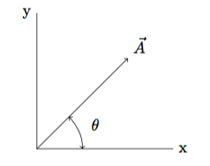Figure 1.

To add or subtract two vectors, it is useful to describe the vector in terms of its x- and y-components. The x-component is the amount of the vector that points in the x-direction, which is mathematically represented as: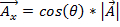. (Equation 1)

The y-component is represented as:. (Equation 2)

The magnitude ofis defined to be: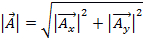. (Equation 3)

To add or subtract two vectors, simply break the vectors down into their x- and y-components and then add or subtract, respectively, the corresponding components.

For example, if vector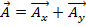and vector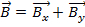, then the addition of the two vectors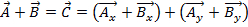.

To determine the angle θ a vector makes with respect to the x-axis, use the following equation: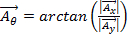. (Equation 4)

Because vectors have both magnitude and direction, multiplying two vectors is not as simple as multiplying two numbers. There are two ways to multiply vectors: the dot product and the cross product. The dot product can be written as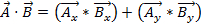or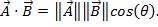Here, θ is the angle between the two vectors. The result only has a magnitude, and not a direction. An application of the dot product in physics is work (W), where work is defined as a force times a distance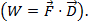The cross product of two vectors can be written as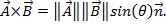While similar to the dot product, the cross product contains the term, which is defined as a vector with magnitude 1 that is perpendicular to the two vectorsand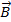. The result of the cross product is a vector. One example of the cross product in physics is torque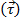, which is the result of a force times a radius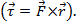Vectors are useful in physics because forces like gravity or friction can be represented as vectors. In this lab, the force of gravity is used to demonstrate the vector nature of forces and how those forces add in multiple directions. The force of gravity on the Earth's surface is written as: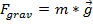, (Equation 5)

where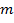is the mass of the object, while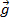is the acceleration of gravity near the Earth's surface (9.8 m/s2).

### Procedure

1. Balance forces.

1. On the force table, set up two pulleys with the same mass facing opposite directions (180° difference in angle).
2. The force of each will be equal to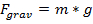. Check whether the two forces are equal and opposite by examining the ring at the center of the force table, which should not move.
3. Notice that if the components of the vectors associated with these forces are added, the resultant vector will have zero magnitude. This is how to determine that all forces are in equilibrium.

2. Analytical calculations.

1. This lab will consist of three forces in equilibrium. Two forces will be known, while the third will be found-first analytically, using the theory of vectors, and then experimentally. For this lab, keepat 0° for the duration.
2. Note that ifandare known and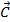, when added to the system, causes the two forces to be in equilibrium, thenis of equal magnitude but in the opposite direction to the sum (+).
3. Calculate the magnitude ofand. Use the fact thatand that 1 Newton (N) is a unit of force equal to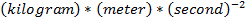.
4. Using the theory of vectors, calculate what magnitudewould be if it was the sum (+).
5. Using the theory of vectors, calculate what anglewould be if it was the sum (+).

3. Experiment.

1. Following the values on the first line of Table 1 forand, set up the two forces on the force table. Remember to keepat 0°.
2. Set up the third force,, by adding weights and changing the angle until equilibrium is reached. Record these values in Table 2.
3. Repeat step 3.2 for each of the four cases.
4. Determine the percent difference from the analytical result by calculating the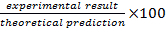. Complete Table 2 with these calculated values.

Vectors are quantities with both magnitude and direction-unlike scalars, which have only a magnitude and sign.

Force, acceleration, and velocity are examples of vectors. While mass, energy, and time, are examples of scalars.

A vector is usually represented by an arrow. The arrow's length corresponds to its magnitude and the angle indicates direction.

This video will show a system of forces that can be analyzed with vector addition and subtraction, and demonstrate how such operations produce results that are important for understanding several physical phenomena.

Describing a vector requires a coordinate system. Within this chosen frame of reference, this example of a ball kicked into the air has an initial velocity vector. As explained earlier, the length of the arrow represents the magnitude of the velocity. And the direction of the vector is its angle from the ground.

Any vector may be decomposed into components, which are vectors themselves along the x- and y-axes. If the ball's initial velocity is 20 meters per second at 60 degrees, the horizontal component is speed times cosine of 60 degrees and has a magnitude of 10 meters per second. The vertical component is speed times sine of 60 degrees and has a magnitude of about 17.3 meters per second.

Vector addition of horizontal and vertical components reconstructs the original velocity vector. To add vectors, imagine placing the head of one to the tail of the other. In this example the vectors happen to be at a right angle. The sum results when traveling directly from the tail of the first to the head of the second.

These components are at a right angle, so the magnitude of the sum is given by the Pythagorean theorem. The angle is the arctangent of the vertical component divided by the horizontal component.

When adding two vectors that are not perpendicular, decompose each into x- and y-components then add corresponding components. Finally, calculate the vector sum of horizontal and vertical components as explained before. Subtracting one vector from another is equivalent to negating the second vector and adding it to the first. As before, decompose each vector into x- and y-components. Then subtract the smaller x-component from the larger one and do the same for y-components. Then, same as before, calculate the vector sum of the resulting x- and y-components.

To demonstrate the addition and subtraction of vectors in a physics lab, the equipment commonly used is a force table. This is a disk with angles marked around the perimeter, a ring in the center attached to cords with masses at the other end suspended by pulleys. The masses produce forces, which are the vectors to be studied. The force along each cord is equal to the gravitational force, or mg, with units of Newtons.

Now, in this set-up, if there are just two equal masses at 180 degrees from each other, then they produce forces with a vector sum of zero. This condition is called equilibrium, which results in zero acceleration and thus the ring won't move.

But if the two forces pulling the ring do not cancel each other, for example due to change in angle, then the non-zero net force would cause the ring to move. In such instances, if we know the magnitudes and directions of these forces, then we can use vector addition and subtraction to calculate the third force needed to re-establish equilibrium.

In the next section we will show how to conduct such force table experiments that test the theoretical principles of vector addition and subtraction

If the two forces are equal and opposite, the ring at the center of the table should not move. In this case, each force vector exactly opposes the other in magnitude and direction. The vector sum has zero magnitude, which is the condition of zero net force, or equilibrium.

To validate the principles of vector addition and subtraction, set up the masses and angles for forces A and B as indicated on the first line of this table. Keep the angle for A at zero degrees. Now, set up the third force by adding masses and changing the angle until the ring does not move.

After achieving equilibrium, calculate the force of C by multiplying its mass by the acceleration due to gravity. Also, record the magnitude and angle for force C.

Repeat this test for the three different cases and record the magnitude and angle of force C each time.

For the four experimental set-ups, this table shows the calculated magnitudes of forces A and B, and angles of B with respect to A. Using the first set-up as an example, we can calculate force C needed to establish equilibrium on the table.

Here force A has a magnitude of 0.98 Newtons at 0°. Force B has the same magnitude of 0.98 Newtons but an angle of 20°. To determine the vector for C, decompose forces A and B into their x- and y-components. Note force A is directed only along the x-axis and has no y-component. Then add the components to yield the x- and y-vectors, which are the sum of A and B vectors.

To achieve equilibrium, the x- and y-components of C must be the opposite of these vectors. To obtain the vector C, move the tail of its y-component to the head of the x-component. Then add the two vectors using the Pythagorean theorem to find the magnitude of vector C. And the angle for C is the arctangent of the vertical component divided by the horizontal component. Therefore, the calculated magnitude of C turns out to be 1.93 Newtons at an angle of 10° with respect to the x-axis.

Now during the experiment, we calculate C through observation and trial and error, by adjusting the weights and angles to prevent motion of the ring on the force table.

And this table shows that experimental and calculated results for both magnitude and angle match closely for all four setups. This agreement validates the representation of forces as vectors. The difference may be attributed to limitations in the accuracy of the weights, measurement accuracy of the angle and unaccounted forces caused by friction on the force table and with the pulleys.

Vector addition and subtraction are used in both simple and complex applications. Let's take a look at some of them.

When touring a city like New York, the distance is usually measured in blocks, and the directions are north, south, east, and west.

A person walking four blocks east and three blocks north undergoes a change in position, which is a vector quantity. Therefore, by applying the equations for vector addition, one can calculate the magnitude and direction of the vector between the start and end points of the walk.

From walking to flying: a pilot is constantly performing mental vector addition and subtraction to maneuver the airplane. By using the wings' flaps and ailerons, a pilot can adjust lift against gravity. If lift is greater than gravitational force, the plane ascends. If lift is less than gravitational force, it descends.

Similarly, a pilot uses the engines to adjust thrust against drag. If thrust is greater than drag, the plane accelerates. If thrust is less than drag, it decelerates.

When the sum of these four forces equals zero, the plane is in equilibrium and cruises at constant speed and altitude.

You've just watched JoVE's introduction to vectors. You should now know how to add and subtract vectors, and understand how certain physical quantities behave as vectors. Thanks for watching!

### Results

The results of the lab are shown in Table 1 and Table 2.

Table 1. Setup.

 Setup # A B Mass Angle Mass Angle 1 100 0 100 20 2 100 0 150 40 3 200 0 150 60 4 200 0 250 80

Table 2. Analytical Results.

 Setup # Magnitude(N) Magnitude(N) Angle(°) Magnitude(N) Angle(°) 1 0.98 0.98 20 1.93 10 2 0.98 1.47 40 2.31 24 3 1.96 1.47 60 2.98 25 4 1.96 2.45 80 3.39 45

Table 3. Experimental Results.

 Setup # Experimental Magnitude(N) Analytical Magnitude(N) Difference (%) Experimental Angle(°) Analytical Angle(°) Difference (%) 1 2.1 1.93 9 11 10 10 2 2.2 2.31 5 26 24 8 3 2.8 2.98 6 28 25 12 4 3.5 3.39 3 43 45 5

The results of the experiment are in agreement with the analytical calculations. The sum of two vectors and the angle between them can be calculated using Equations 1-5. The equations are valid for making calculations of physical vectors, such as force.

### Applications and Summary

An outfielder in baseball has to understand vectors in order to catch a ball on the move. If the outfielder only knew the speed of the ball, he might run to leftfield instead of to right and miss the ball. If he only knew the direction of the hit, he might charge in, only to watch the ball sail over his head. If he understands vectors, then as soon as the ball is hit, he can consider both the magnitude and direction in order to estimate where the ball is going to be when he makes a catch.

When an airplane is in the sky, its speed and direction can be written as a vector. When there is a heavy wind, the wind vector adds to the vector of the plane to give the resultant system vector. For example, if a plane is flying into the wind, the magnitude of the resultant vector will be less than the initial magnitude. This corresponds to the plane moving slower when heading into the wind, which makes intuitive sense.

When two objects collide and stick together, their final momentum (a vector) can be approximated as the sum of the two initial momentum vectors. This is a simplification, as in the real world, two objects colliding have extra factors to consider, like heat or deformation from the collision. Momentum is just the mass of an object multiplied by its velocity. If two skaters on ice traveling in different directions and at different speeds collide and hold onto each other, their final direction and speed can be estimated based upon their initial vector components.

In this experiment, the vector nature of forces was examined and measured. Vectors were added together, and the resultant magnitude and direction were determined both analytically and experimentally.X